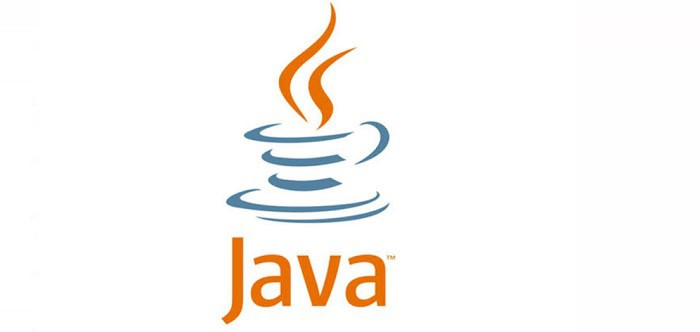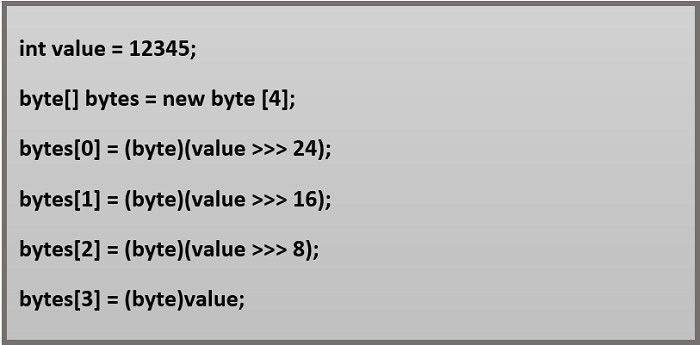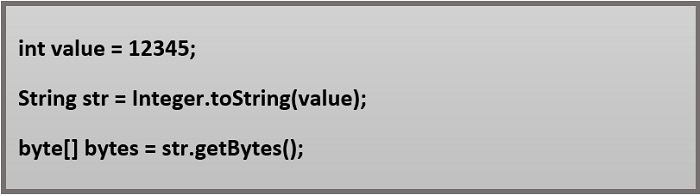# Java to Convert Int to Byte Array: Efficient Coding TechniquesJava provides several ways to convert an int to a byte array. This article will explore some known techniques and discuss their pros and cons.First, let’s start by explaining what a byte array is. A byte array, also known as a byte buffer or byte sequence, can store data of any type, such as integers, characters, and floating-point numbers. In Java, a byte array is represented by the byte[] data type, an array of bytes with a length of 8 bits each.

## How To Use Java To Convert An Int To A Byte Array

We can use Java to convert an int to a byte array in many ways. Some of those are listed below:### Using The ByteBuffer Class

One of the simplest and most efficient ways to convert an int to a byte array is by using the ByteBuffer class. This class provides a convenient way to allocate, store, and manipulate a buffer of bytes.

To convert an int to a byte array, we can use the following code:In this example, we create a ByteBuffer object with a capacity of 4 bytes, which is enough to store an int value. Then, we use the putInt() method to store the int value in the buffer. Finally, we use the array() method to retrieve the byte array from the buffer.

The advantage of using the ByteBuffer class is that it provides a convenient and efficient way to manipulate byte arrays. It can also handle the system’s endianness (the order in which the bytes are stored).

### Using the Integer Class

Another way to convert an int to a byte array is by using the Integer class, which provides several methods to perform this conversion.

To convert an int to a byte array, we can use the following code:In this example, we use the same technique as before, but we use the allocate() and putInt() methods of the ByteBuffer class to store the int value in a buffer, and then we use the array() method to retrieve the byte array.

The advantage of using the Integer in java is that it provides several conversion methods and is easy to use.

### Using The Bitwise Operators

We can also use the bitwise operators to convert an int to a byte array, which allows us to manipulate the individual bits of a value.

To convert an int to a byte array, we can use the following code:In this example, we create a byte array with a length of 4, and then we use the bitwise right shift operator (>>>) to extract the individual bytes of the int value. Finally, we store the bytes in the byte array.

The advantage of using the bitwise operators is that it provides a low-level and efficient way to manipulate the individual bits of a value.

### Using The toString() and getBytes() Methods

The fourth way to convert an int to a byte array in Java is by using the toString() and getBytes() methods.

To convert an int to a byte array, we can use the following code:In this example, we first use the toString() method of the Integer class to convert the int value to a string. Then, we use the getBytes() method of the String class to convert the string to a byte array.

The advantage of using the toString() and getBytes() methods is that it is simple and easy to understand. However, the disadvantage is that it may not be as efficient as the other methods, especially for large values.

## FAQs

### What happens in Java when we convert an int to a byte?

Java removes the first 24 bytes from an integer value before converting it to a byte. Bitwise AND will be used to hide all of the extra sign bits. Here is a Java program example that transforms an integer into a byte.

### Why not swap out int for byte?

Sending a byte rather than an int will save you space and bandwidth if you're undertaking network programming. Even 4 bytes might be included in an int.

### Should you choose byte over int?

When dealing with big arrays, the byte data type might be helpful when memory savings are crucial. They can also replace it when their constraints make your code more understandable; a variable's range restriction can operate as documentation.

### Can an integer store in a byte?

Integers from 0 up to 4,294,967,295 (232 - 1) may store because words of memory usually used to store integers are 4 bytes or 32 bits in size. The integers 1 through 5 are represented below as four-byte values, with each row corresponding to one integer.

### Which is quicker, bytes or ints?

Since 32-bit numbers are more productive for CPUs, ints are often quicker. The main purpose of using bytes would be to save memory. Using big files, live data, etc.

### Can Java be used to convert a byte array to a file?

We will use the getBytes() function of the String class to convert a byte array to a file. Implementation: Create a byte array from a String and write it to a file. Consider Java.

### Why does a byte matter?

Most computers represent a character, such as a letter, number, or typographic sign, using a unit called a byte. A string of bits that must be utilized in a bigger unit for application reasons can store in each byte. Software that displays graphics, for instance, can create a visual image from a stream of data.

### In Java, why do we utilize the byte data type?

Since a byte is much smaller than an int, it can be beneficial when building big data arrays. Bytes are helpful for file modification and reading since it is possible to read files byte-by-byte using various ways. The user input can also be read byte-by-byte as an alternative.

### How does memory store a byte?

Digital information is store in units called bytes, typically eight bits. The byte is the lowest accessible memory unit in various computer systems since it was historically the number of bits needed to represent a single text character in a computer.

### How many bytes of data may store?

A byte is a collection of eight bits. It can hold 256 distinct values because it has 8 bits to work with. This makes it suitable for storing a single text since we can give each letter, numeral, punctuation mark, etc., a separate 8-bit pattern using 256 different values.

## Conclusion

There are various ways to convert an int to a byte array in Java, each with pros and cons. The best method to use depends on your specific requirements and the constraints of your application. Some of the most popular techniques are using the ByteBuffer class, the Integer class, the bitwise operators, and the toString() and getBytes() methods.